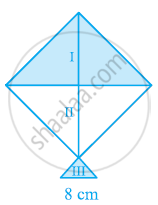Share

# A Kite in the Shape of a Square with a Diagonal 32 cm and an Isosceles Triangles of Base 8 Cm and Sides 6 cm Each is to Be Made of Three Different Shades as Shown in the Given Figure. How Much Paper of Each Shade Has Been Used in It? - CBSE Class 9 - Mathematics

ConceptApplication of Heron’S Formula in Finding Areas of Quadrilaterals

#### Question

A kite in the shape of a square with a diagonal 32 cm and an isosceles triangles of base 8 cm and sides 6 cm each is to be made of three different shades as shown in the given figure. How much paper of each shade has been used in it?#### Solution

We know that

Area of square = 1/2(diagonal)2

"Area of the given kite "= 1/2(32 cm)^2 = 512 cm^2

Area of 1st shade = Area of 2nd shade = 512/2 = 256 cm2

Therefore, the area of paper required in each shape is 256 cm2.

For IIIrd triangle

Semi-perimeter,

s=(6+6+8)/2=10 cm

By Heron’s formula,

"Area of triangle "=sqrt(s(s-a)(s-b)(s-c))

"Area of 3rd triangle "=sqrt(10(10-6)(10-6)(10-8))

=(sqrt(10xx4xx4xx2))cm^2

=(4xx2sqrt5)cm^2

=8sqrt5 cm^2

= (8 x 2.24) cm2

= 17.92 cm2

Area of paper required for IIIrd shade = 17.92 cm2

Is there an error in this question or solution?

#### APPEARS IN

NCERT Solution for Mathematics Class 9 (2018 to Current)
Chapter 12: Heron's Formula
Ex. 12.20 | Q: 7 | Page no. 207

#### Video TutorialsVIEW ALL 

Solution A Kite in the Shape of a Square with a Diagonal 32 cm and an Isosceles Triangles of Base 8 Cm and Sides 6 cm Each is to Be Made of Three Different Shades as Shown in the Given Figure. How Much Paper of Each Shade Has Been Used in It? Concept: Application of Heron’S Formula in Finding Areas of Quadrilaterals.
S